## Aptitude :: Alligation Or MixturePage 1

1. Alligation:
It is the rule that enables us to find the ratio in which two or more ingredients at the given price must be mixed to produce a mixture of desired price.

2. Mean Price:
The cost of a unit quantity of the mixture is called the mean price.

3. Rule of Alligation:
If two ingredients are mixed, then
Quantity of cheaper/Quantity of dearer = C.P. of dearer - Mean Price/QMean price - C.P. of cheaper

∴ (Cheaper quantity) : (Dearer quantity) = (d - m) : (m - c).

4. Suppose a container contains x of liquid from which y units are taken out and replaced by water.
After n operations, the quantity of pure liquid = [x(1 - y/x)n]units.

1.

 In what ratio must rice at Rs. 9.30 per kg be mixed with rice at Rs. 10.80 per kg so that the mixture be worth Rs. 10 per kg ? (a)7 : 8 (b)8 : 7 (c)9 : 7 (d)7 : 9
Answer is: BBy the rule of alligation, we have:Required ratio = 80 : 70 = 8 : 7.

2.

 How much water must be added to 60 litres of milk at 1( Â½) litres for Rs. 20 So as to have a mixture worth Rs.10(2/3) a litre ? (a)12 litre (b)13 litre (c)14 litre (d)15 litre
Answer is: DC.P. of 1 litre of milk = Rs. (20 x 2/3) = Rs. 40/3Ratio of water and milk = 8/3 : 32/3 = 8 : 32 = 1 : 4
Quantity of water to be added to 60 litres of milk = [1/4 x 60 ]litres =15 lt.

3.

 In what ratio must water be mixed with milk to gain 20% by selling the mixture at cost price? (a)1 : 5 (b)5 : 1 (c)3 : 5 (d)5 : 3
Answer is: ALet C.P. of milk be Re. 1 per litre.
Then, S.P. of 1 litre of mixture = Re. 1.
Gain obtained = 20%.
C.P. of 1 litre of mixture = Rs.[(100/120) x 1]=Rs.5/6
By the rule of alligation, we have:Ratio of water and milk = 1/6 : 5/6 = 1 : 5

4.

 The milk and water in two vessels A and B are in the ratio 4 : 3 and 2: 3 respectively. In what ratio, the liquids in both the vessels be mixed to obtain a new mixture in vessel C containing half milk and half water? (a)5 : 7 (b)7 : 5 (c)6 : 7 (d)7 : 6
Answer is: BLet the C.P. of milk be Re. 1 per litre
Milk in 1 litre mixture of A = 4/7 litre
Milk in 1 litre mixture of B = 2/5 litre
Milk in 1 litre mixture of C = Â½ litre
C.P. of 1 litre mixture in A = Re .4/7
C.P. of 1 litre mixture in B = Re.2/5
Mean price = Re.1/2
By the rule of alligation, we have:Required ratio = 1/10 : 1/14 = 7 : 5

5.

 In what proportion must rice at Rs. 3.10 per kg be mixed with rice at Rs. 3.60 per kg so that the mixture be worth Rs. 3.25 per kg ? (a)7 : 3 (b)7 : 5 (c)7 : 6 (d)5 : 7
Answer is: ABy the rule of alligation, we have: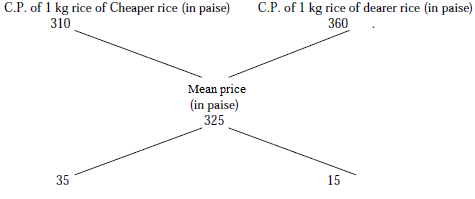By the allegation rule:
(Quantity of cheaper rice) / (Quantity of dearer rice) = 35/15 = 7/3
They must be mixed in the ratio 7 : 3.

6.

 How many kilograms of sugar costing Rs. 6.10 per kg must be mixed with 126 kg of sugar costing Rs. 2.85 per kg so that 20% may be gained by selling the mixture at Rs. 4.80 per kg? (a)67 kg (b)68 kg (c)66 kg (d)69 kg
Answer is: DS.p of one Kg of mixture = Rs. 4.80, Gain = 20%.
C.P of one kg of mixture = Rs. [(100/120) x 4.80] = Rs. 4.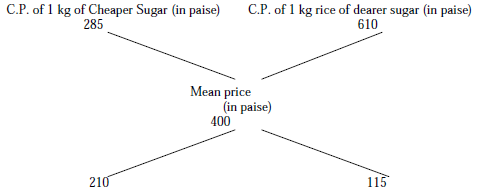(Quantity of cheaper sugar)/(Quantity of dearer sugar) = 210/115.
(Quantity of cheaper sugar)/(Quantity of dearer sugar) = 42/23.
If cheaper sugar is 42 kg, dearer one = 23 kg
If cheaper sugar is 126 kg,
Dearer one = [(23/42) x 126]kg= 69 kg.

7.

 A butler stole wine from a butt of sherry which contained 40% of spirit and he replaced what he had stolen by wine containing only 16% spirit. The butt was then of 24% strength only. How much of the butt did he steal? (a)2/3 (b)4/5 (c)6/5 (d)3/2
Answer is: A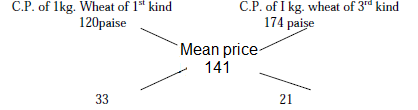By allegation rule:
(Wine with 40% spirit) / (Wine with 16% spirit) = 8/16 = 1/2.
i.e., they must be mixed in the ratio (1 : 2).
Thus 1/3 of the butt of sherry was left and hence the butler drew out 2/3 of the butt.

8.

 In what ratio must a person mix three kinds of wheat costing him Rs. 1.20,Rs. 1.44 and Rs. 1.74 per kg., so that the mixture may be worth Rs. 1.41 per kg? (a)7 : 8 : 9 (b)11 : 77 : 7 (c)12 : 88 : 9 (d)11 : 88 : 7
Answer is: BStep 1.
Mix wheats of first and third kind to get a mixture worth Rs. 1.41 per kg.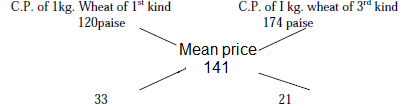By allegation rule :
(Quantity of 1st kind of wheat)/(Quantity of 3rd kind of wheat) = 33/21
(Quantity of 1st kind of wheat)/(Quantity of 3rd kind of wheat) = 11/7
i.e., they must be mixed in the ratio 11 : 7.
Step 2.
Mix wheats of first and second kind to get a mixture worth Rs. 1.41 per kg.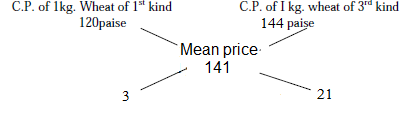By alligation rule :
(Quantity of 1st kind of wheat) / (Quantity of 3rd kind of wheat) = 3/21
(Quantity of 1st kind of wheat) / (Quantity of 3rd kind of wheat) = 1/7
i.e., they must be mixed in the ratio 1 : 7.
Thus, (Quantity of 2nd kind of wheat)/(Quantity of 1st kind of wheat) = [(Quantity of 2nd kind of wheat) x (Quantity of 1st kind of wheat)]/[(Quantity of 1st kind of wheat) x (Quantity of 3rd kind of wheat)] = [(7/1) x (11/7)] = 11/1.
Quantities of wheat of(1st kind : 2nd kind : 3rd kind) = (1 : 7 : 7/11).
Quantities of wheat of(1st kind : 2nd kind : 3rd kind) = (11 : 77 : 7).

No comment yet.

### More subjects

copyright 2014-2018 This site is powered by sNews | Login